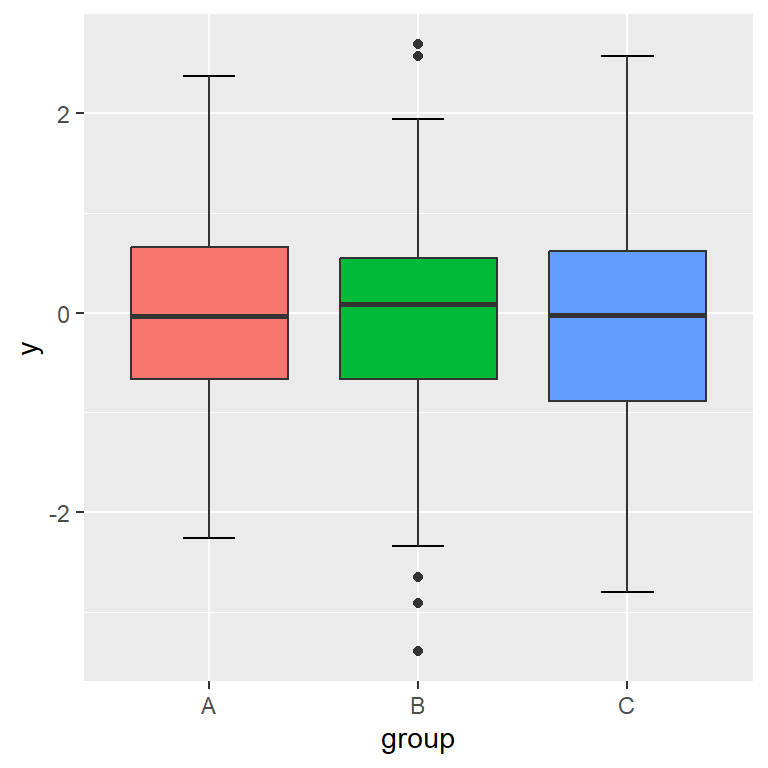# Box plot by group in ggplot2

## Sample data

In this tutorial we are going to use the following data frame, which first column is as numerical variable and the second a categorical variable representing groups.

``````# Sample dataset
set.seed(136)
df <- data.frame(y = rnorm(300),
group = sample(LETTERS[1:3],
size = 300,
replace = TRUE))``````

## Box plot by group with `geom_boxplot`

In order to create a basic grouped box plot in R you need to pass the variables to `aes` and use the `geom_boxplot` geom as in the following example.

``````# install.packages("ggplot2")
library(ggplot2)

# Box plot by group
ggplot(df, aes(x = group, y = y)) +
geom_boxplot()``````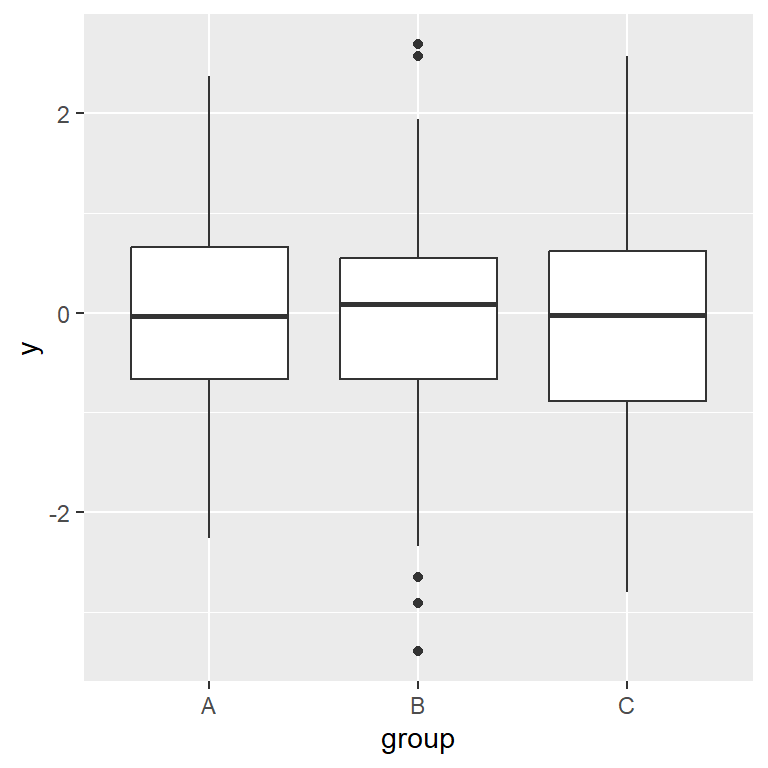## Adding error bars with `stat_boxplot`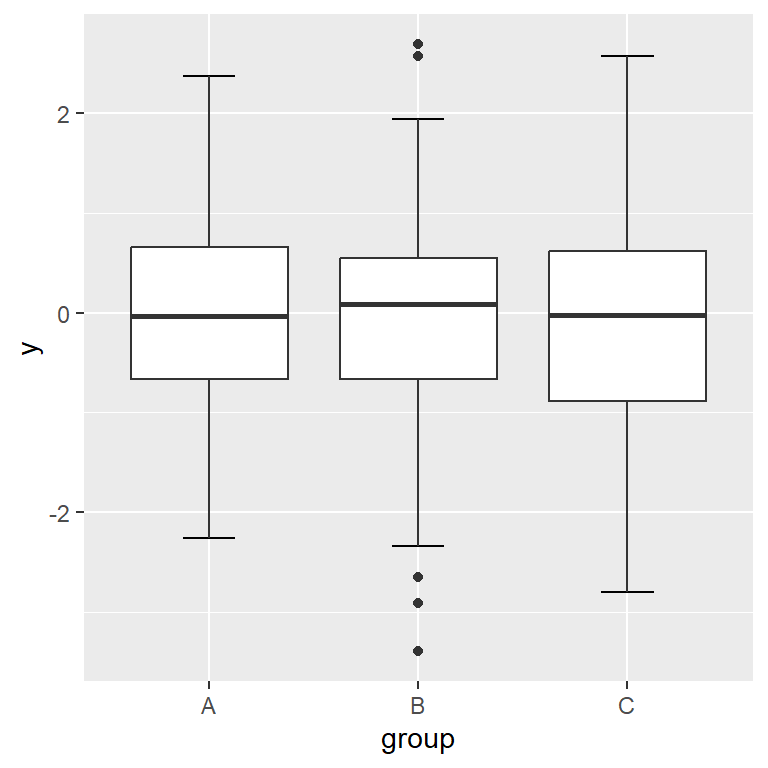As you may have noticed, the default box plot in ggplot2 doesn’t add error bars. If you want to add them use the `stat_boxplot` stat and set `geom = "errorbar"`. The width of the bars can be customized with `width` argument.

``````# install.packages("ggplot2")
library(ggplot2)

# Box plot by group with error bars
ggplot(df, aes(x = group, y = y)) +
stat_boxplot(geom = "errorbar", # Error bars
width = 0.25) +    # Bars width
geom_boxplot()``````

## Horizontal box plot by group

The box plots can also be displayed in horizontal or landscape mode. To accomplish it you can change the order of your variables inside `aes` or use `coord_flip`, as shown above.

Option 1: change the order of the variables

Set the grouping variable on the Y-axis.

``````# install.packages("ggplot2")
library(ggplot2)

# Horizontal box plot in ggplot2
ggplot(df, aes(x = y, y = group)) +
stat_boxplot(geom = "errorbar",
width = 0.25) +
geom_boxplot()``````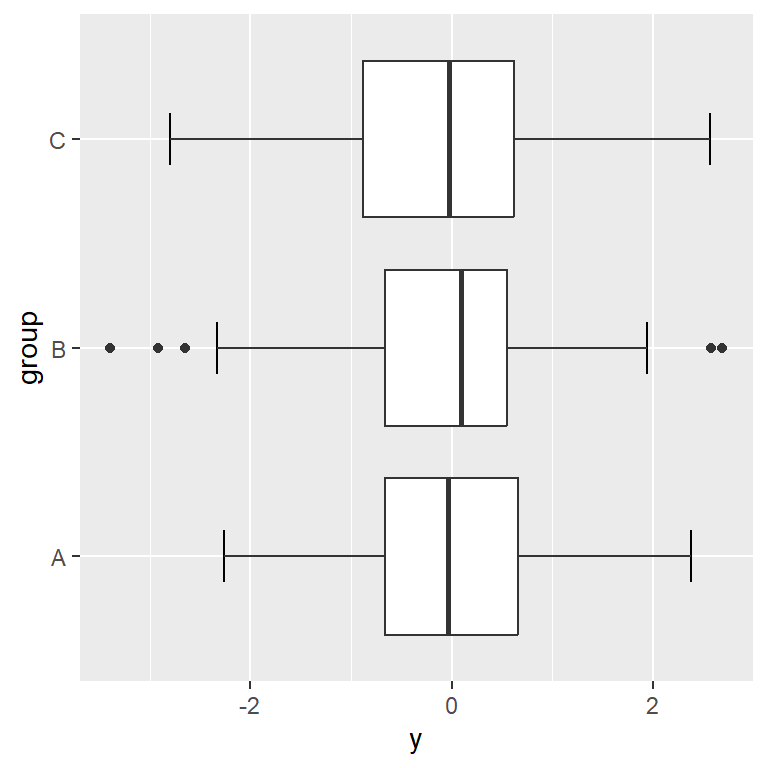Option 2: use `coord_flip`

`coord_flip` will invert the axes, so a vertical box plot will become horizontal and viceversa.

``````# install.packages("ggplot2")
library(ggplot2)

# Horizontal box plot
ggplot(df, aes(x = group, y = y)) +
stat_boxplot(geom = "errorbar",
width = 0.25) +
geom_boxplot() +
coord_flip()``````## Color customization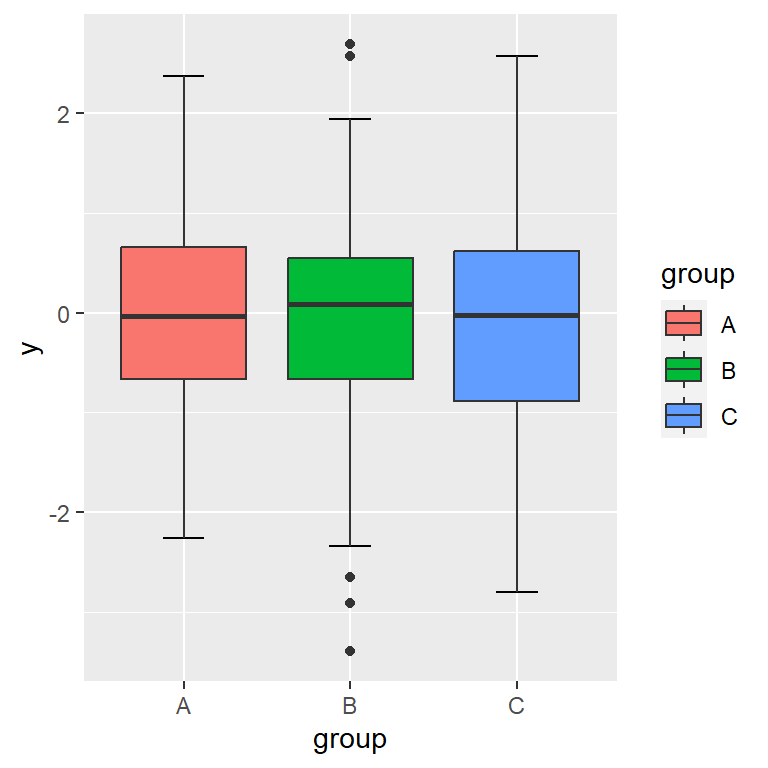If you pass the categorical variable to the `fill` argument of `aes`, each box plot will be filled with a color and a legend will be displayed.

``````# install.packages("ggplot2")
library(ggplot2)

ggplot(df, aes(x = group, y = y, fill = group)) +
stat_boxplot(geom = "errorbar",
width = 0.25) +
geom_boxplot()``````

The colors or the box plots are fully customizable. In the following example we are setting a fill color for each group, changing the border color of the boxes and setting the color of the outliers to black.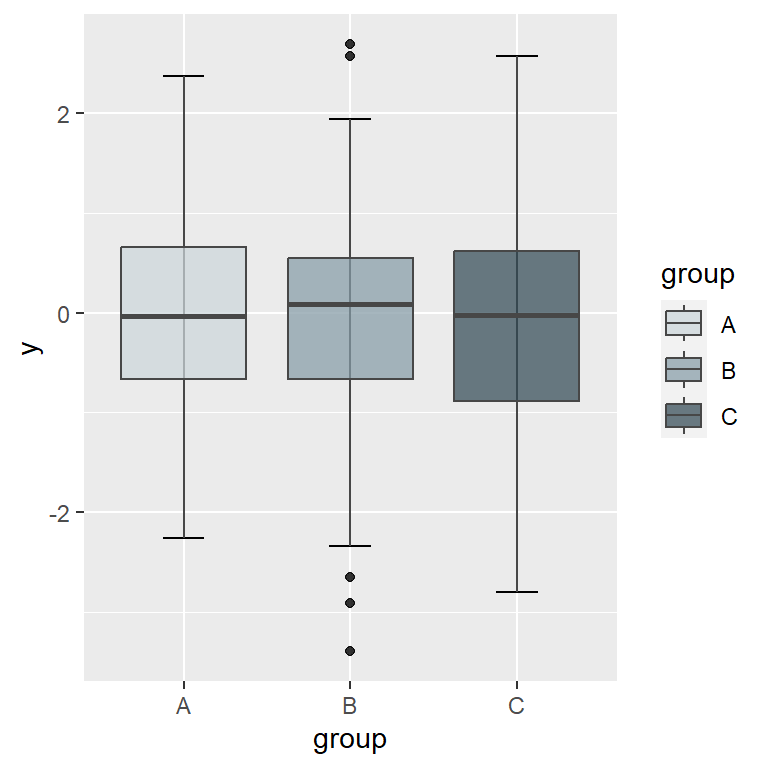``````# install.packages("ggplot2")
library(ggplot2)

# Fill colors
cols <- c("#CFD8DC", "#90A4AE", "#455A64")

ggplot(df, aes(x = group, y = y, fill = group)) +
stat_boxplot(geom = "errorbar",
width = 0.25) +
geom_boxplot(alpha = 0.8,          # Fill transparency
colour = "#474747",   # Border color
outlier.colour = 1) + # Outlier color
scale_fill_manual(values = cols)   # Fill colors``````

## Legend customization

Change the title

You can change the default title of the legend with `guides` function as follows.

``````# install.packages("ggplot2")
library(ggplot2)

ggplot(df, aes(x = group, y = y, fill = group)) +
stat_boxplot(geom = "errorbar", width = 0.25) +
geom_boxplot() +
guides(fill = guide_legend(title = "Title"))``````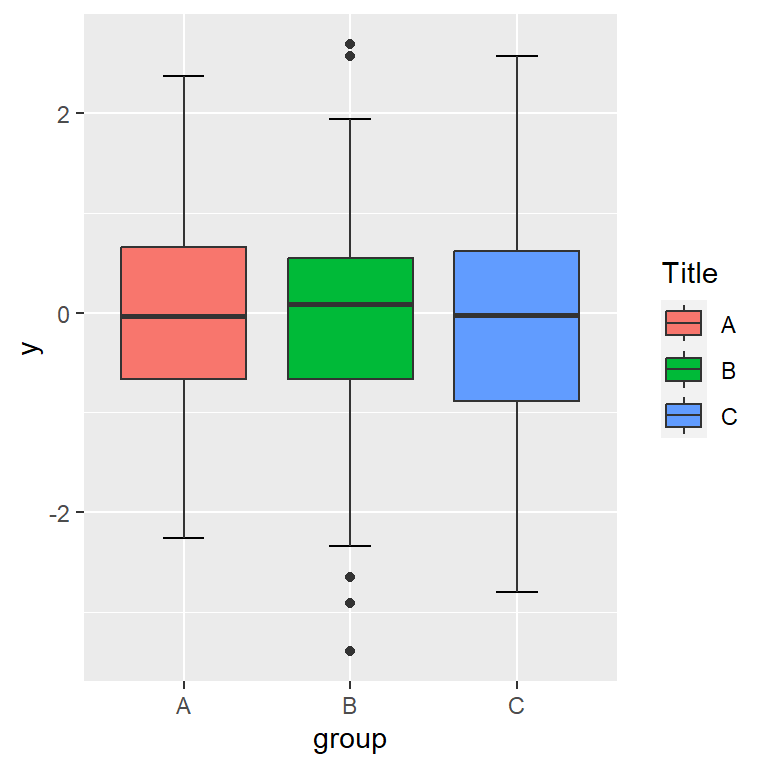Change the labels

The legend labels are the levels of the categorical variable. You can override them with `scale_fill_hue`.

``````# install.packages("ggplot2")
library(ggplot2)

ggplot(df, aes(x = group, y = y, fill = group)) +
stat_boxplot(geom = "errorbar", width = 0.25) +
geom_boxplot() +
scale_fill_hue(labels = c("G1", "G2", "G3"))``````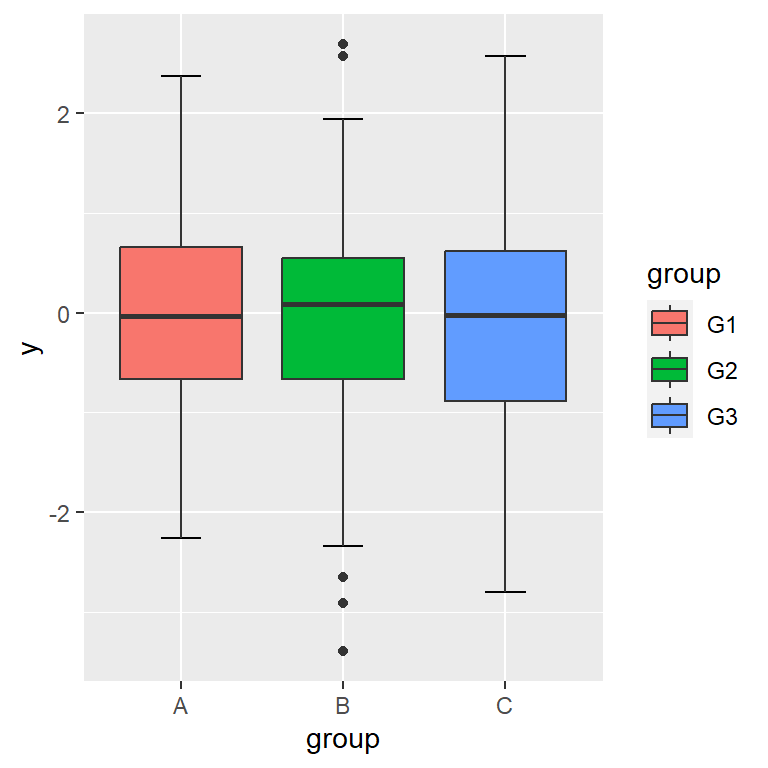Remove the legend

If you don’t want any legend displayed you can remove it with `theme(legend.position = "none")`.

``````# install.packages("ggplot2")
library(ggplot2)

ggplot(df, aes(x = group, y = y, fill = group)) +
stat_boxplot(geom = "errorbar", width = 0.25) +
geom_boxplot() +
theme(legend.position = "none")``````